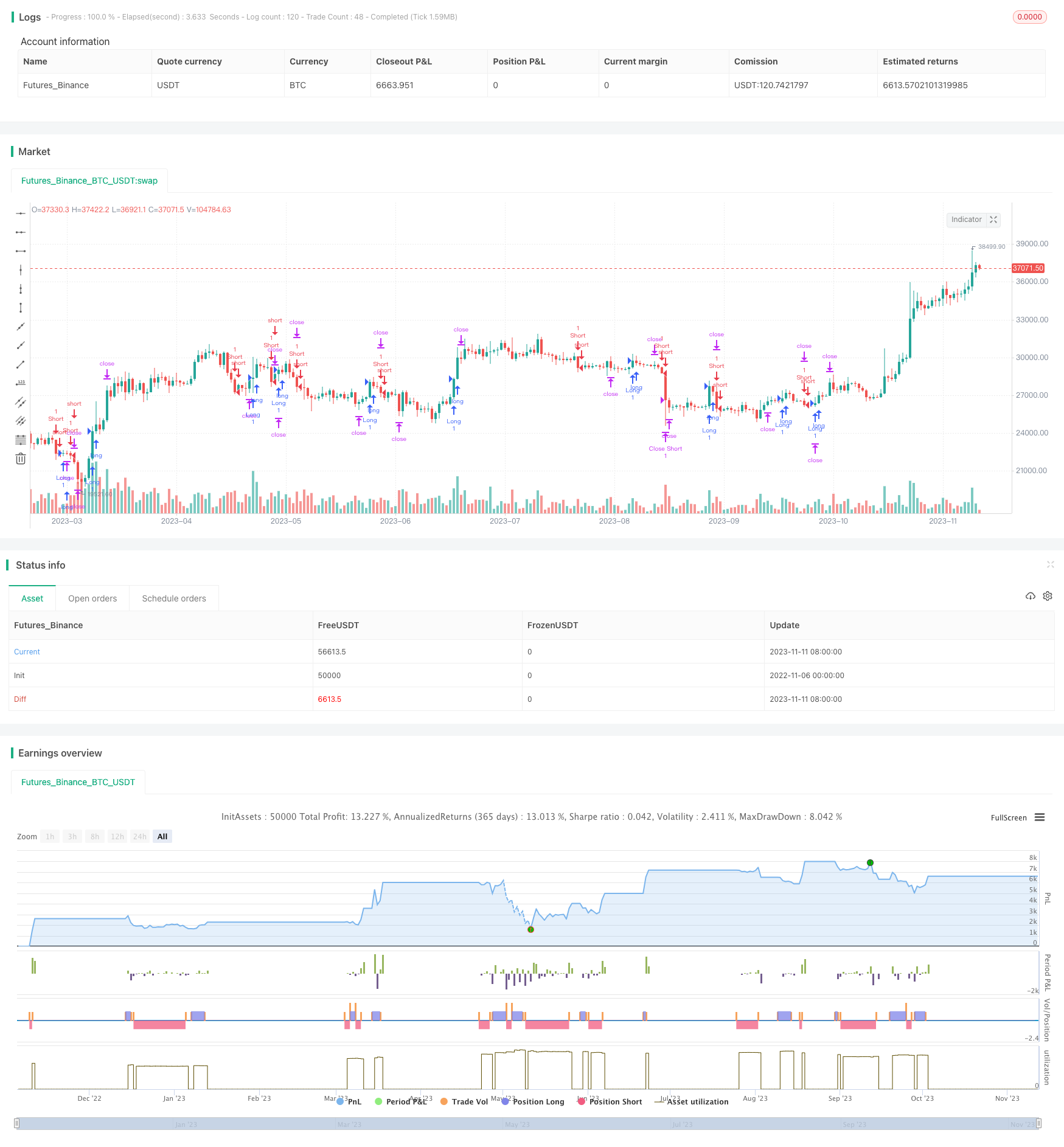# Dual DI Crossover Daily Trading Strategy

Author: ChaoZhang, Date: 2023-11-13 11:32:08
Tags:## Overview

This strategy generates trading signals based on the crossover of the positive directional indicator (DI+) and negative directional indicator (DI-) calculated from the average true range (ATR). It belongs to the trend following strategy that identifies trend reversal points through the crossover of DI+ and DI-. ATR is used to set stop loss and take profit levels.

## Strategy Logic

1. Calculate ATR(14): Compute the average true range over the past 14 days using high, low and close prices.

2. Compute DI+ and DI-:

• DI+ = 100 * RMA(MAX(UP,0),N) / ATNR

• DI- = 100 * RMA(MAX(DOWN,0),N) / ATNR

Where UP is the difference between current high and previous close, DOWN is the difference between current low and previous close, N is the parameter period, default to 14, and ATNR is the ATR calculated from step 1.

3. Determine entry and exit:

• When DI+ crosses over DI-, a buy signal is generated.

• When DI+ crosses below DI-, a sell signal is generated.

4. Set stop loss and take profit:

• Long stop loss is entry price minus ATR multiplied by the stop loss multiplier

• Long take profit is entry price plus ATR multiplied by the take profit multiplier

• Short stop loss is entry price plus ATR multiplied by the stop loss multiplier

• Short take profit is entry price minus ATR multiplied by the take profit multiplier

1. Using DI+/DI- crossover to determine trend reversal provides timely signal for new trend direction.

2. ATR as dynamic stop loss/take profit indicator can set reasonable levels based on market volatility.

3. The strategy has few parameters and is easy to understand and implement.

4. Backtest results show this strategy has positive profit factor and outperforms buy & hold.

## Risks and Solutions

1. False signal risk from DI crossover

• Filter signals with moving averages etc. to avoid false breakout.
2. Stop loss/take profit too close

• Adjust ATR multiplier to accommodate volatility.
3. Ineffective in range-bound market

• Combine with other indicators to filter signals in consolidation.
4. Drawdown risk

• Drawdown is acceptable but unavoidable for systematic strategies. Adjust position sizing to control drawdown.

## Optimization Suggestions

1. Add filters like moving average to avoid false signals in range-bound periods.

2. Implement position sizing like fixed fractional or Martingale to control drawdown and boost profitability.

3. Optimize ATR parameters to match volatility of different trading instruments.

4. Parameter optimization on DI period, ATR period, ATR multiplier etc. to find optimum combination.

## Conclusion

This is a simple and practical strategy generating signals from DI crossover and setting dynamic stop loss/take profit with ATR. With few parameters, it is easy to test and optimize. But DI crossover is less effective during consolidation. Going forward, combining additional filters is the main improvement area. Overall this strategy demonstrates stable performance suitable for short-term day trading.

```/*backtest
start: 2022-11-06 00:00:00
end: 2023-11-12 00:00:00
period: 1d
basePeriod: 1h
exchanges: [{"eid":"Futures_Binance","currency":"BTC_USDT"}]
*/

// This source code is subject to the terms of the Mozilla Public License 2.0 at https://mozilla.org/MPL/2.0/
//@version=4
strategy("DI Crossing Daily Straregy HulkTrading", overlay=true)
// ATR Multiplier. Recommended values between 1..4
atr_multiplier = input(1, minval=1, title="ATR Multiplier")
//Length of DI. Recommended default value = 14
length = input(14, minval=1, title="Length di")
up = change(high)
down = -change(low)
range = rma(tr, 14)

//DI+ and DI- Calculations
di_plus = fixnan(100 * rma(up > down and up > 0 ? up : 0, length) / range)
di_minus = fixnan(100 * rma(down > up and down > 0 ? down : 0, length) / range)

//Long and short conditions
longCond = crossover(di_plus,di_minus)
shortCond = crossunder(di_plus,di_minus)

//Stop levels and take profits
stop_level_long = strategy.position_avg_price - atr_multiplier*atr(14)
take_level_long = strategy.position_avg_price + 2*atr_multiplier*atr(14)
stop_level_short = strategy.position_avg_price + atr_multiplier*atr(14)
take_level_short = strategy.position_avg_price - 2*atr_multiplier*atr(14)

//Entries and exits
strategy.entry("Long", strategy.long, when=longCond)
strategy.exit("Close Long","Long", stop=stop_level_long, limit = take_level_long)
strategy.entry("Short", strategy.short, when=shortCond)
strategy.exit("Close Short","Short", stop=stop_level_short, limit = take_level_short)

```

More